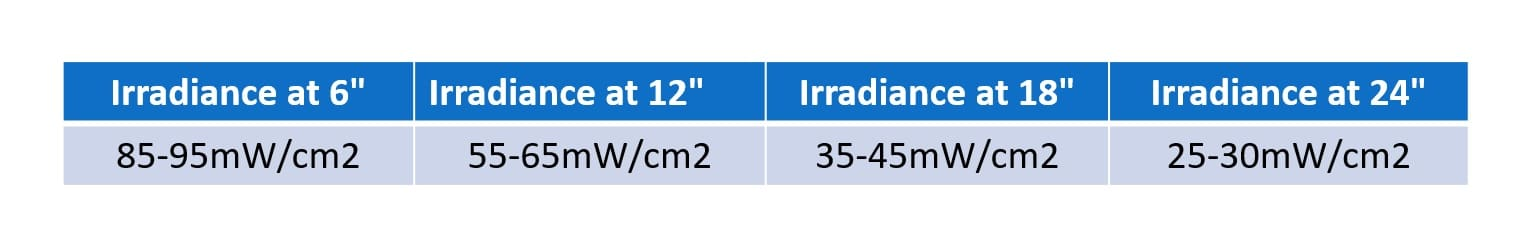# Red Light Therapy Dosing Guide

If you want an effective light therapy session, you must have an effective dose. That requires:

• A light that is relatively powerful (i.e. has an ideal “power density”)
• Ideally, a light that can treat a large area of the body at once
• An understanding of the optimal duration of time using the light to get the right total dose
Light therapy dose is calculated with this formula:

Power Density x Time = Dose

Fortunately, most recent studies use standardised units to describe their protocol:

• Power Density in mW/cm² (milliwatts per centimeter squared)
• Time in (seconds)
• Dose in J/cm² (Joules per centimeter squared)

Too little of a dose and you get minimal to no effects. Too strong of a dose and you get minimal to no effects.

Let’s talk about power density of the light first.

Most studies showing benefits of red/NIR light therapy used light outputs of 20-200mW/cm2.

This is basically a measurement of power density – how much power the light is emitting (in watts) over how big of an area.

To put that in different terms, if you shine the light on your body (let’s say, for the sake of ease of calculation, that it’s an area of 50cm x 40cm, which equals 2,000cm2)…

And the light you’re using is 200 watts (which is 200,000mW), then you have 200,000mW/2,000cm2 = 100mW/cm2

That’s a great power density.

This is presented in an excessively simple way for the sake of clarity. In reality, there are factors that make this calculation much more complex, like the fact that actual wattage differs from claimed wattage for most lights, and the distance away from the light dramatically changes the power density, among other factors.

Overall, the device needs to emit light above a certain power density (light intensity), needs to be at the right wavelengths, be at the proper distance away from your body, and ideally, needs to be physically large enough to emit light over a large portion of your body.

But for simplicity, let’s leave all these nuances of the calculations out of it.

The next part of the equation is how long should you apply the light. The dose (duration of exposure) is calculated by:

## 200.000mW / 2000cm² = 100mW/cm²

Dose = Power Density x Time

So all we are doing is taking that number we already have (mW/cm2) and then the “dose” can be calculated once you know how long you should apply that light for. Here’s the equation you need to calculate the dose:

mW/cm2 x time (in seconds) x 0.001 = J/cm2

Here’s the critical piece of information you need to know: The dose you want to shoot for is between 3J/cm2 – 50J/cm2.

Depending on whether you’re treating superficial areas like the skin or surface wounds or deeper tissues like muscles/organs, etc., you want different doses.

Here are some sample calculations to show you how this works:

• 20mW/cm² applied for 50 seconds gives 1J/cm².
• 50mW/cm² applied for 20 seconds gives 1J/cm²
• 75mW/cm² applied for 15 seconds gives 1J/cm²
• 100mW/cm² applied for 10 seconds gives 1J/cm²

These units of mW/cm² and seconds give a result in mJ/cm² – just multiply that by 0.001 to get in J/cm². The full formula, taking into account standard units is therefore:

Dose = Power Density x Time x 0.001Now you might be wondering, “Okay, so how do I know whether to use it for 1 minute or 10 minutes? And how do I know whether to use it from 6” away or 24” away?”

Good questions!

For skin issues (e.g. anti-aging benefits) and other more superficial (near to the surface) body issues, there are a few things to note. We want a relatively low overall dose on each area of skin, of roughly 3-15J. Also, there is some indication that lower power densities (below 50mW/cm2) may actually be more optimal for treating the skin than very higher power densities.

In contrast, for treating deep tissues, you want bigger doses and higher power density (light intensity) for optimal effects. You want doses of 10-60J. So in general, you’d want to have the light much closer to your body with a much higher light intensity. That’s what’s needed to deliver optimal doses of light deep into your tissues.

To sum up: With skin/surface treatments, you want to be further away from the light which lowers the light intensity and covers a broader area of your body for an overall lower dose. With deeper tissues, you want to be closer to the light which increases the light intensity for an overall higher dose.